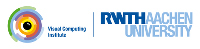OpenMesh
Using Smart Handles

This examples shows:

• How to use Smart Handles and ranges to navigate on the mesh
• How to use Smart Ranges

So far we have used methods such as halfedge_handle(), next_halfedge_handle(), prev_halfedge_handle(), oppopsite_halfedge_handle(), face_handle(), to_vertex_handle(), and some others, to navigate on that mesh. These functions are defined on a mesh and require as input a handle to an element of the mesh, such as VertexHandle or HalfedgeHandle. In the following example we iterate over all vertices of a triangle mesh and for each vertex we create a list of the vertices that lie opposite of the edges in the ring around the vertex:

// iterate over vertices of the mesh
for (auto vh : mesh.vertices())
{
std::vector<OpenMesh::VertexHandle> opposite_vertices;
// iterate over all outgoing halfedges
for (auto heh : mesh.voh_range(vh))
{
// navigate to the opposite vertex and store it in the vector
opposite_vertices.push_back(mesh.to_vertex_handle(mesh.next_halfedge_handle(mesh.opposite_halfedge_handle(mesh.next_halfedge_handle(heh)))));
}
}

For a more concise way of navigating OpenMesh provides smart handles, OpenMesh::SmartVertexHandle, OpenMesh::SmartHalfedgeHandle, OpenMesh::SmartEdgeHandle, and OpenMesh::SmartFaceHandle. Smart handles are smart, because they know to which mesh they belong. This allows them to provide functions for navigating the mesh allowing us to write the above code much simpler:

// iterate over vertices of the mesh
for (auto vh : mesh.vertices())
{
// iterate over all outgoing halfedges
std::vector<OpenMesh::VertexHandle> opposite_vertices;
for (auto heh : vh.outgoing_halfedges())
{
// navigate to the opposite vertex and store it in the vector
opposite_vertices.push_back(heh.next().opp().next().to());
}
}

The ranges of OpenMesh that are returned by functions like voh_range() or outgoing_halfedges() all provide a few methods than can simplify some calculations (see OpenMesh::SmartRangeT). One example is the to_vector() method which convertes the range of elements into a vector containing the elements. All of these methods take a functor as argument (sometimes optional) which is called for each element of the range. With this, the above code can also be implemented like this:

// iterate over vertices of the mesh
for (auto vh : mesh.vertices())
{
// create lambda that returns opposite vertex
auto opposite_vertex = [](OpenMesh::SmartHalfedgeHandle heh) { return heh.next().opp().next().to(); };
// create vector containing all opposite vertices
auto opposite_vertices = vh.outgoing_halfedges().to_vector(opposite_vertex);
}
Definition: SmartHandles.hh:170

## Code Example

In this example, we will use bi-laplacian smoothing on a mesh. We store the `laplace` vector which is the vector pointing from a vertex to the center of gravity of its neighboring vertices in a vertex property.

// Add a vertex property storing the laplace vector
auto laplace = OpenMesh::VProp<MyMesh::Point>(mesh);
This class is intended to manage the lifecycle of properties.
Definition: PropertyManager.hh:76

To compute the center of gravity, i.e. the average position, we use the avg() method of the range of 1-ring vertices and pass in a PropertyManager acting as functor returning the corresponding point of a vertex.

// Get a propertymanager of the points property of the mesh to use as functor
auto points = OpenMesh::getPointsProperty(mesh);
// Smooth the mesh several times
for (int i = 0; i < iterations; ++i) {
// Iterate over all vertices to compute laplace vector
for (const auto& vh : mesh.vertices())
laplace(vh) = vh.vertices().avg(points) - points(vh);

Similarily we compute the update vector as the laplace of the freshly computed laplace vectors by simply exchanging the points property manager with the laplace property manager.

// Iterate over all vertices to compute the laplace vector of the laplace vectors
for (const auto& vh : mesh.vertices())
bi_laplace(vh) = (vh.vertices().avg(laplace) - laplace(vh));

Finally, we apply the update after damping it by a factor of -0.5.

// update points by substracting the bi-laplacian damped by a factor of 0.5
for (const auto& vh : mesh.vertices())
points(vh) += -0.5 * bi_laplace(vh);

Below is the complete source code:

#include <OpenMesh/Core/IO/MeshIO.hh>
#include <OpenMesh/Core/Mesh/DefaultTriMesh.hh>
#include <OpenMesh/Core/Utils/PropertyManager.hh>
#include <iostream>
#include <vector>
int main(int argc, char** argv)
{
MyMesh mesh;
if (argc != 4) {
std::cerr << "Usage: " << argv << " #iterations infile outfile" << std::endl;
return 1;
}
const int iterations = argv;
const std::string infile = argv;
const std::string outfile = argv;
std::cerr << "Error: Cannot read mesh from " << infile << std::endl;
return 1;
}
{
// Add a vertex property storing the laplace vector
auto laplace = OpenMesh::VProp<MyMesh::Point>(mesh);
// Add a vertex property storing the laplace of the laplace
auto bi_laplace = OpenMesh::VProp<MyMesh::Point>(mesh);
// Get a propertymanager of the points property of the mesh to use as functor
auto points = OpenMesh::getPointsProperty(mesh);
// Smooth the mesh several times
for (int i = 0; i < iterations; ++i) {
// Iterate over all vertices to compute laplace vector
for (const auto& vh : mesh.vertices())
laplace(vh) = vh.vertices().avg(points) - points(vh);
// Iterate over all vertices to compute the laplace vector of the laplace vectors
for (const auto& vh : mesh.vertices())
bi_laplace(vh) = (vh.vertices().avg(laplace) - laplace(vh));
// update points by substracting the bi-laplacian damped by a factor of 0.5
for (const auto& vh : mesh.vertices())
points(vh) += -0.5 * bi_laplace(vh);
}
} // The laplace and update properties are removed from the mesh at the end of this scope.
// Write mesh file
std::cerr << "Error: Cannot write mesh to " << outfile << std::endl;
return 1;
}
}
bool read_mesh(Mesh &_mesh, const std::string &_filename)
Read a mesh from file _filename.
Definition: MeshIO.hh:95
Triangle mesh based on the ArrayKernel.
Definition: TriMesh_ArrayKernelT.hh:96
Polygonal mesh based on the ArrayKernel.
Definition: PolyMesh_ArrayKernelT.hh:96

Project OpenMesh, ©  Visual Computing Institute, RWTH Aachen. Documentation generated using doxygen .